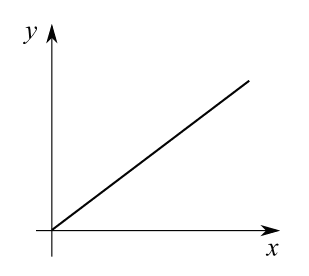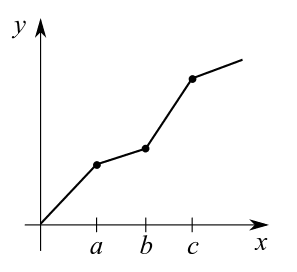# Calculation of the delta time when changing the tempo

17
Undo
How is the delta time calculated when the tempo changes? My program works fine until there are multiple tempo changes between two notes that have a very long silence, the problem is noticeable in multitrack mode and it starts to desynchronize.
one part of the ticks is with the first tempo and the other part of ticks are the new tempo?

Tempo Change (FF 51 03) = 545440, Delta Time: 0
//... more noteon and noteoff events
NoteOn [0x99, 36, 100] Delta: 398
NoteOff [0x89, 36, 0] Delta: 85
NoteOn [0x99, 36, 100] Delta: 275
NoteOff [0x89, 36, 0] Delta: 72
Tempo Change (FF 51 03) = 526300 Delta: 196057
Tempo Change (FF 51 03) = 521710 Delta: 458
Tempo Change (FF 51 03) = 517240 Delta: 3100
Tempo Change (FF 51 03) = 512820 Delta: 1552
Tempo Change (FF 51 03) = 508460 Delta: 1428
Tempo Change (FF 51 03) = 504200 Delta: 1117
Tempo Change (FF 51 03) = 500000 Delta: 843
NoteOn [0x99, 36, 100] Delta: 21520
NoteOff [0x89, 36, 100] Delta: 20
1 month ago
1
Undo
Not sure what you're doing here. The data you show may be out of context. Certainly, important information is missing.

It would be more help if you showed the problem in the form of a Standard Midi File.

Due to the missing into, I'm making a total guess.

The note data would relate to specific tracks/channels. Although the tempo data could be in any channel, it will relate to all notes/events in all channels. To keep this clear, and avoid confusion, it is usual to place all tempo change instructions in a separate track, and not mix them with other things.

In effect, there are two separe timing processes running live during playback of a midi file. One is the 'real time' ticking of the computer clock. The other is the formula that converts this time into the musical tick count. Each time you implement a Tempo Change, this second value is modified so that the tempo changes as needed. Maybe you've got this happening one way in one channel, a different way in another channel?

Again, seeing the complete midi file would help.

Geoff
1 month ago
0
Undo
I did a simple example attached, the problem occurs on the 2nd track when changing to 50 bmp (2nd track gets out of sync with 1st and ends after, this does not happen if there are no silences), my suspicion is that the stored delta ticks of the 9th quarter note are influenced by the previous bmp of 300
1 month ago
1
Undo
Delta times are just a mechanism to save space in the file; they are not necessary to understand how the timing works. Just use absolute tick numbers.

All events occur at a specific time, measured in ticks. The length of a tick can vary, as controlled by tempo change events. The tempo change event itself occurs at a specific absolute tick number; ticks before this event have the old length, and all following ticks have the new length.

In all tracks, the same (absolute) tick number occurs at the same time. If a tempo change event occurs between two events in another track, then there is no easy mechanism to compute the time between these events at once; the easiest way to handle this is to merge all tracks (at least for timing calculations).
1 month ago
1
Undo
Here is my understanding of your problem:

When you play the MIDI file you attached in a particular MIDI player, the notes in the second track begin to go out of sync with the notes in the first track. As part of trying to understand what is causing the problem, you want to understand how to manually convert a position in absolute ticks to a position in absolute seconds.

How to convert ticks to seconds

As Clemens Ladisch said, you should first convert delta ticks into absolute ticks. In other words, instead of using the tick position from the previous event in the track (delta ticks), calculate the tick position from the beginning (absolute ticks). You do this by adding all the delta ticks in the particular track you are concerned about up to and including the delta ticks for the event you are concerned about.

Next, to convert the amount of ticks into an amount of quarter notes, use the "ticks per quarter note" resolution specified in the MIDI file. In the MIDI file you attached, the resolution is 480 ticks per quarter note. So divide the amount of ticks by 480 to get the amount of quarter notes.

Next, as you know, each tempo event in a MIDI file is actually a conversion factor in microseconds per quarter note.

To convert an amount of quarter notes into an amount of microseconds....

First let's start with an example where there is only one tempo for the entire file:x is the amount of quarter notes from the beginning
y is the amount of microseconds from the beginning
m is the conversion factor in microseconds per quarter note

y = m x

Now let's look at an example where there are multiple tempos in the file:x is amount of quarter notes from the beginning
y is amount of microseconds from the beginning

m0 is the conversion factor in microseconds per quarter note from the beginning to x=a
ma is the conversion factor in microseconds per quarter note from x=a to x=b
mb is the conversion factor in microseconds per quarter note from x=b to x=c
mc is the conversion factor in microseconds per quarter note from x=c to the end

 if x is between 0 and a: y = m0 x if x is between a and b: y = m0 a + ma (x - a) if x is between b and c: y = m0 a + ma (b - a) + mb (x - b) if x is between c and the end: y = m0 a + ma (b - a) + mb (c - b) + mc (x - c)
1 month ago
0
Undo
Thanks, now I understand my problem, I thought that all delta time ticks were calculated based on the last tempo change.PS: I modified my code and it WORKS Thanks to all.
1 month ago
0
Undo
I understand that you've sorted out your code.

Just for information.

I've looked at your midi file in one of my systems, specifically the 'Piano Roll' display. All the notes display totally reasonably within the bars, and all appear to be totally in sync with other notes, regardless of tempo changes, tracks, whatever. So there does not appear to be any problem.

I attach a little text file, a text display of your midi file. This process shows all the Delta Times (Ticks) as accumulating numbers as other replies above suggested. This may clarify things?

Geoff
1 month ago
0
Undo
I attach a little text file, a text display of your midi file. This process shows all the Delta Times (Ticks) as accumulating numbers as other replies above suggested. This may clarify things?

Counting the number of accumulated ticks was how I solved it, I used that file to verify that I correctly calculated the accumulated ticks, and it gives me exactly the same thing. Thank you
• Page :
• 1
There are no replies made for this post yet.
Be one of the first to reply to this post!